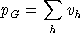An Algebraic Statement of the Samuelson Model

Zero Net Profit for Private Production
The unit cost of production in sector As is given by a nested Leontief - Cobb-Douglas function defined over the cost of intermediate inputs and primary factors with ad-valorem taxes on factor demands. Unlike the Harberger models, tax rates in this model are determined endogenously. In equilibrium, the unit cost must be no less than the market price of output: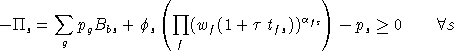Zero Net Profit for Public Sector Contractors
The unit cost of public provision is determined by the market price of commodity inputs to the Leontief activity. Input requirements are defined by a vector of public sector input coefficients, ag. In equilibrium, the price paid by the government equals the cost of market inputs: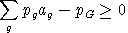Income Balance for Government
Government tax income (PT) is determined by the value of tax revenue, calculated using activity levels, compensated demands, market prices and ad-valorem tax rates. In equilibrium, the value of tax revenue equals the market cost of public sector output: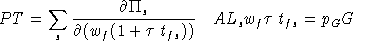Income Balance for Households
Household income is determined by the net of tax return to primary factors plus the imputed value of public provision: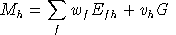Market Clearance for Private Goods
Producer output is equal to the sum of intermediate plus final demand: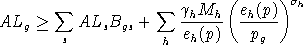where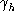is the household budget share devoted to the consumption of goods, and eh is the "unit expenditure function" which may be written: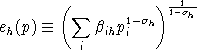Personalized Markets for Public Goods
We assume a "pure" public good in this model, hence each household may attach a different marginal valuation to public provision in an equilibrium. In order to compute these marginal values, we include a separate public good "market" for each houshold which balances the level of provision with the household "demand":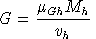.

in which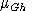is the budget share of public goods in the top-level Cobb-Douglas preferences of household h.

Market Clearance for Factors
The aggregate supply of factors equals the sum of producer and consumer demand. Producers pay taxes on factor inputs, consumers do not because we consider these demands to be "leisure" or "household production". Consumer demands for factors are specified as Cobb-Douglas (constant budget shares):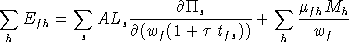Samuelson Rule for "Optimal" Provision of Public Goods
The tax rate multiplier is adjusted to balance the marginal cost of public provision with the summation across households of marginal willingness to pay. Due to the existence of household factor demand, factor taxes are necessarily distortionary and there will be an excess social cost of public funds. For this reason, the Samuelson rule is neither necessary nor sufficient for optimal provision. We apply the rule here merely to illustrate the programming methodology, even though the resulting equilibrium may be "suboptimal":